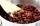# Mass + weighted average - problems

1. CoffeeIn stock are three kinds of branded coffee prices: I. kind......248 Kč/kg II. kind......134 Kč/kg III. kind.....270 Kč/kg Mixing these three species in the ratio 10:7:7 create a mixture. What will be the price of 1100 grams of this mixture?
2. Vinegar 2How many percentage of getvinegar solution, if we mix to 2 liters of 5.9% and 2 liters of 3.5% vinegar?
3. Center of gravityThe mass points are distributed in space as follows - specify by coordinates and weight. Find the center of gravity of the mass points system: A1 [14; -2; 5] m1 = 10.2 kg A2 [-2; -16; 7] m2 = 13.6 kg A3
4. CandiesThere are two types of candies in the store at 20 CZK/kg and 80 CZK/kg. How much will be cost a mix if we mix 22kg cheaper and 4kg more expensive candies?
5. Dried fruitThe manufacturer produces a mixture of dried fruit. He purchased: 10kg pineapple for 200 Kc/kg 2kg papaya for 180 kc/kg 1kg of banana for 400 Kc/kg How many kgs of raisin for 80 Kc/kg must be put into the mix by the manufacturer so that the production pr
6. CuSO4 mixtureHow many grams of solid CuSO4 we have to add to 450g of 15% CuSO4 solution to produce a 25% solution?
7. PeroxideHow many ml 30% of peroxide (H2O2) should be poured into 100ml H2O to give a 20% solution?
8. Centroid - two bodiesA body is composed of a 0.8 m long bar and a sphere with a radius of 0.1m attached so that its center lies on the longitudinal axis of the bar. Both bodies are of the same uniform material. The sphere is twice as heavy as the bar. Find the center of gravit
9. Mixture 2How many liters of water must be added to 7 liters of a 20% solution to obtain a 10% solution?

We apologize, but in this category are not a lot of examples.
Do you have an interesting mathematical problem that you can't solve it? Enter it, and we can try to solve it.

To this e-mail address, we will reply solution; solved examples are also published here. Please enter e-mail correctly and check whether you don't have a full mailbox.